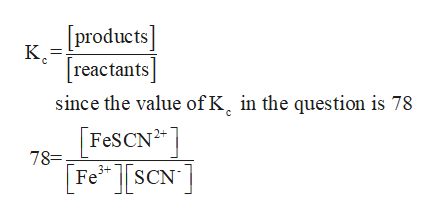# In Part C, we look at the following reaction:Fe3+ (aq) + SCN- (aq) ⇆ FeSCN2+ (aq)a. Write the equilibrium constant expression for the reaction if the equilibrium constant is 78.b. Does the equilibrium mixture contain more products or reactants?c. If more SCN- is added to the equilibrium mixture, will the red color of the mixture intensify or lessen? Explain.

Question
107 views

In Part C, we look at the following reaction:

Fe3+ (aq) + SCN- (aq) ⇆ FeSCN2+ (aq)

a. Write the equilibrium constant expression for the reaction if the equilibrium constant is 78.

b. Does the equilibrium mixture contain more products or reactants?

c. If more SCN- is added to the equilibrium mixture, will the red color of the mixture intensify or lessen? Explain.

check_circle

Step 1

a)The given reaction is

Step 2

Equilibrium constant is ratio of concentration of products and reactants.help_outlineImage Transcriptionclose[products] reactants since the value of K, in the question is 78 FESCN2 78= FeSCN 3+ fullscreen
Step 3

b)   Since the value of Kc is 78, it means that the reaction has almost reached completion. If Kc is much greater than 1 then it m...

### Want to see the full answer?

See Solution

#### Want to see this answer and more?

Solutions are written by subject experts who are available 24/7. Questions are typically answered within 1 hour.*

See Solution
*Response times may vary by subject and question.
Tagged in

### Equilibrium Concepts